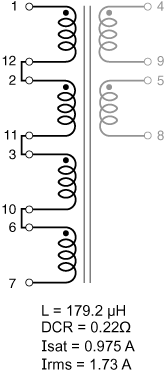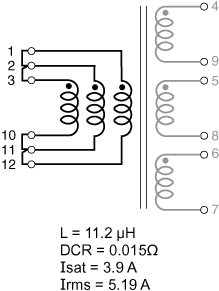## 사이트 설정

##### 통화 설정
제품
RF
EMI
자동차용
리소스
제품 설명서
취급 및 가공
모델 및 레이아웃 도구
교육
일반
비디오 라이브러리
Cx Family Common Mode Chokes
0402CT Low Profile Chip Inductors
XAL7050 High-inductance Shielded Power Inductors
XGL4020 Ultra-low DCR Power Inductors
품질
품질 인증
소재 인증
안전 인증
신뢰성
취급/가공
정보
정보
채용
위치
리소스

# Application example

for Hexa-Path – Inductors

<- Back

## Formulas used to calculate electrical characteristics

Connecting windings in series:

• Inductance = Inductance(table) × (number of windings)2
• DCR = DCR(table) × number of windings
• Isat = (Isat(table) × 6) ÷ number of windings connected in series
• ​​Irms = Irms(table)

Connecting windings in parallel:

• Inductance = Inductance(table)
• DCR = 1 ÷ [number of windings × (1 ÷ DCR(table))]
• Isat = (Isat(table) × 6) ÷ number of windings connected in series
• Irms = Irms(table) × number of windings

### Inductors – using multiple windings

Part number Inductance (µH) Percent Tolerance DCR max (Ω) Volt-time product (V-µs) Peak energy storage (µJ) Isat (A) Irms (A)
HP3-0138L 11.2 20 0.055 30.4 1.656 0.65 1.73

Connecting windings in series:
For higher inductance, the windings (Wn) can be connected in series. As inductance increases, energy storage and Irms remain the same, but DCR increases and Isat decreases.

Example: Calculate new electricals for HP3-0138L with four windings (Wn) connected in series:
• Inductance = Inductance(table)× Wn2 = 11.2 × 42 = 179.2 μH
• DCR = DCR(table)  × Wn = 0.055 × 4 = 0.22 Ohms
• Isat = (Isat(table)  × 6) ÷ Wn = (0.65 × 6) ÷ 4 = 0.975 A
• Irms = Irms(table) = 1.73 AConnecting windings in parallel:
To increase current ratings, the windings (Wn) can be connected in parallel. DCR decreases, current ratings increase, and inductance remains the same.

Example: Calculate new electricals for HP5-0083L, with three (Wn) windings connected in parallel (equivalent to one winding in series):
• Inductance = Inductance(table) = 11.2 μH
• DCR = 1 ÷ [Wn × (1 ÷ DCR(table))] = 1 ÷ [3 × (1 ÷ 0.045)] = 0.015 Ohms
• Isat = (Isat(table) × 6) ÷ Wn = (0.65 × 6) ÷ 1 = 3.9 A
• Irms = Irms(table) × Wn = 1.73 × 3 = 5.19 A